Question

# Find the mass of urea (CH4N2O) needed to prepare 51.3 g of a solution in water...

Find the mass of urea (CH4N2O) needed to prepare 51.3 g of a solution in water in which the mole fraction of urea is 7.66×10−2.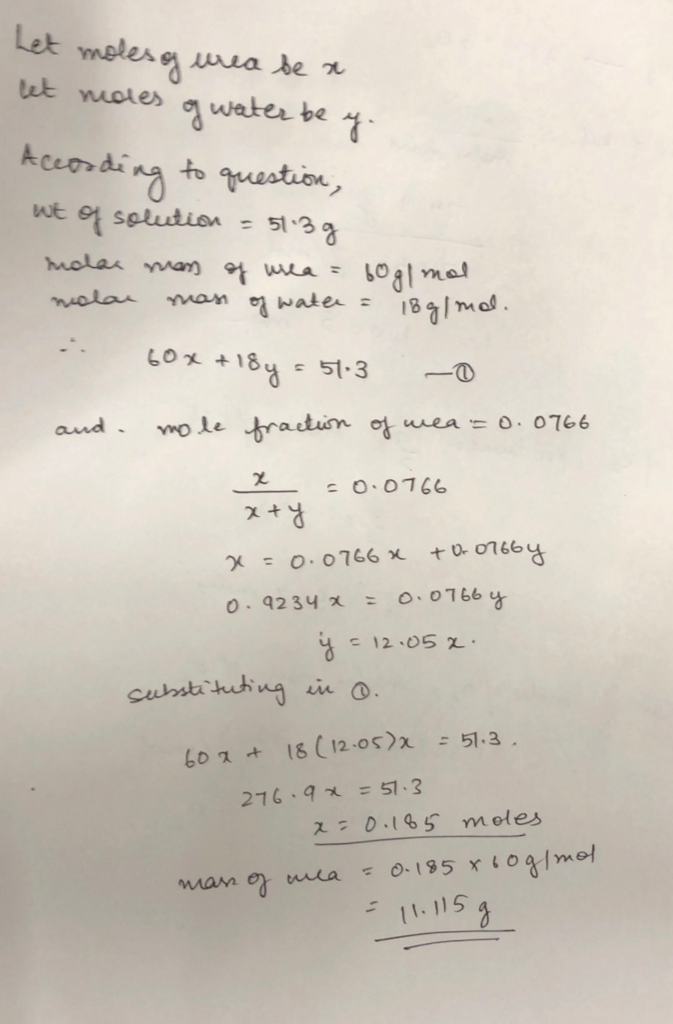#### Earn Coins

Coins can be redeemed for fabulous gifts.

Similar Homework Help Questions
• ### Find the mass of urea (CH4N2O) needed to prepare 51.3 g of a solution in water...

Find the mass of urea (CH4N2O) needed to prepare 51.3 g of a solution in water in which the mole fraction of urea is 7.53×10−2.

• ### 7. What is the mole fraction of urea, CH4N2O, in an aqueous solution that is 46% urea by mass? 8. What volume of a...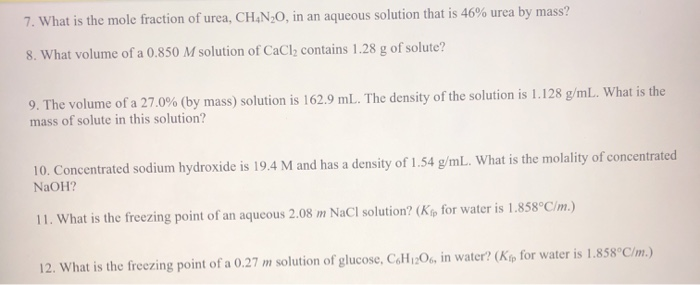7. What is the mole fraction of urea, CH4N2O, in an aqueous solution that is 46% urea by mass? 8. What volume of a 0.850 M solution of CaCl2 contains 1.28 g of solute? 9. The volume of a 27.0% (by mass) solution is 162.9 mL. The density of the solution is 1.128 g/mL. What is the mass of solute in this solution? 10. Concentrated sodium hydroxide is 19.4 M and has a density of 1.54 g/mL. What is the...

• ### what is the mole fraction of urea, CH4N2O, in an aqueous solution that is 59% urea...

what is the mole fraction of urea, CH4N2O, in an aqueous solution that is 59% urea by mass?

• ### The vapor pressure of an aqueous solution of urea, CH4N2O, is 291.2 mm Hg. The vapor...

The vapor pressure of an aqueous solution of urea, CH4N2O, is 291.2 mm Hg. The vapor       pressure of water at this temperature is 355.1 mm Hg. Calculate the mole fraction of urea in       this solution. a. 0.180 b. 0.220 c. 0.500 d. 0.820 e. 1.22 please show all work

• ### The industrially important chemical, urea [CH4N2O; molar mass = 60.0 grams per mole], is prepared from...

The industrially important chemical, urea [CH4N2O; molar mass = 60.0 grams per mole], is prepared from the reaction of ammonia [NH3: molar mass = 17.0 grams per mole] with carbon dioxide [CO2; molar mass = 44.0 grams per mole] according to the following: 2 NH3 + CO2  CH4N2O + H2O If one reacts 132.0 grams of CO2 with 136.0 grams of ammonia, which of these would be the limiting reagent?

• ### What mass of water must be used to prepare a solution of 25.5 g of CaCl2...

What mass of water must be used to prepare a solution of 25.5 g of CaCl2 dissolved in water if the mole fraction of the CaCl2 in solution is 0.105?

• ### 0. 000 45) The concentration of urea in a solution prepared by dissolving 16 g of...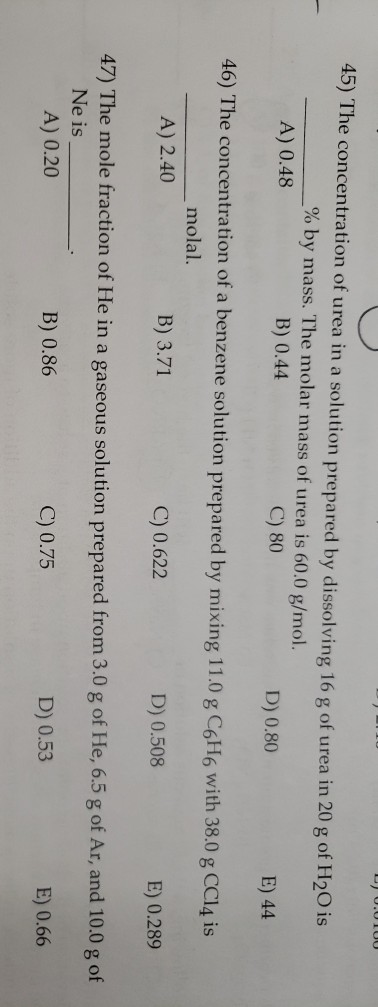0. 000 45) The concentration of urea in a solution prepared by dissolving 16 g of urea in 20 g of H2O is _% by mass. The molar mass of urea is 60.0 g/mol. A) 0.48 B) 0.44 C) 80 D) 0.80 E) 44 46) The concentration of a benzene solution prepared by mixing 11.0 g C6H6 with 38.0 g CCl4 is molal. A) 2.40 B) 3.71 C) 0.622 D) 0.508 E) 0.289 47) The mole fraction of He in...

• ### a solution was prepared by dissolving 27.0 g KOH in 100 g water. A) what is...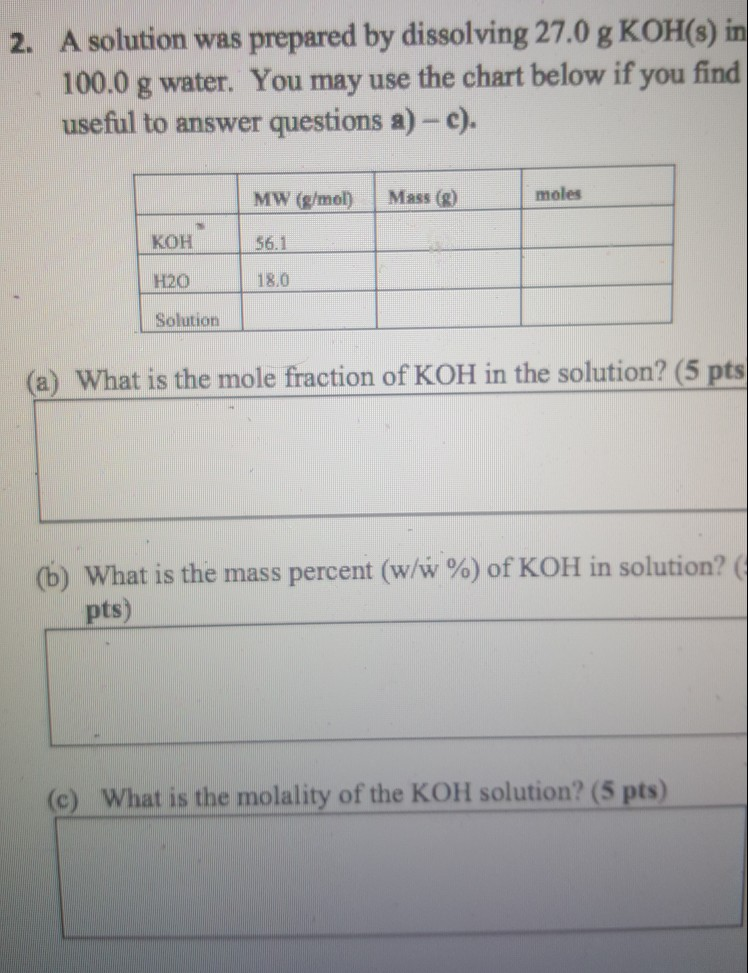a solution was prepared by dissolving 27.0 g KOH in 100 g water. A) what is mole fraction of KOH in solution? B) what is mass percent?C) what is the molality? 2. A solution was prepared by dissolving 27.0 g KOH(s)in 100.0 g water. You may use the chart below if you find usefiul to answer questions a) -e) MW (g/mol Mass ()moles 56.1 H20 18.0 Solution (a) What is the mole fraction of KOH in the solution? (5 pts...

• ### How many grams of water are needed to prepare 250. g of a solution that is...

How many grams of water are needed to prepare 250. g of a solution that is 5.00% by mass NaCl?

• ### 1) What mass of water is needed to dissolve 43.5 g of copper(II) chloride to prepare...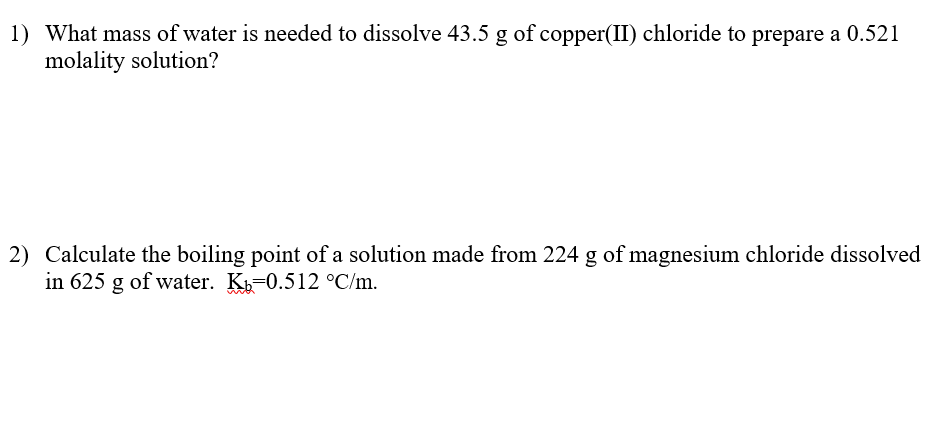1) What mass of water is needed to dissolve 43.5 g of copper(II) chloride to prepare a 0.521 molality solution? 2) Calculate the boiling point of a solution made from 224 g of magnesium chloride dissolved in 625 g of water. Kb=0.512 °C/m.